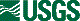## Conversion Factors

For readers who wish to convert measurements from the metric system of units to the inch-pound system, the conversion factors are listed below.

 Multiply By To obtain Length millimeter 0.039 inch centimeter 0.394 inch meter 3.281 foot kilometer 0.621 mile Area square kilometer 0.386 square mile Volume liter 0.264 gallon cubic meter 1.308 cubic yard

A simple rule of thumb for approximate conversions: 1 inch equals about 25 millimeters, or about 2.5 centimeters; 1 yard equals about 1 meter; 1 quart equals about 1 liter.